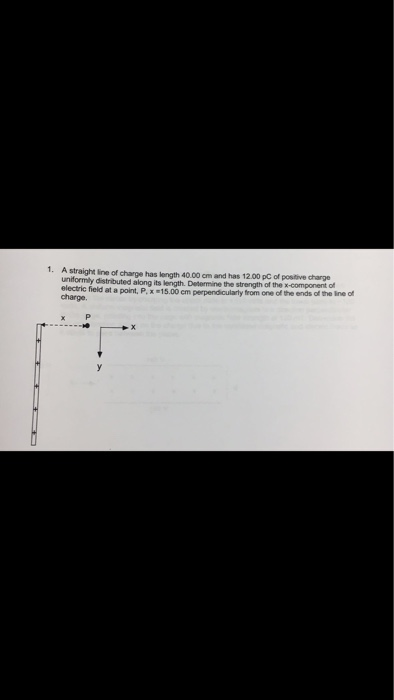# A straight line of charge has length 40.00 cm and has 12.00 pC of positive charge...

###### Question:A straight line of charge has length 40.00 cm and has 12.00 pC of positive charge uniformly distributed along its length. Determine the strength of the x-component of electric field at a point, P, x = 15.00 cm perpendicularly from one of the line of charge.

#### Similar Solved Questions

##### Sam Strother and Shawna Tibbs are vice presidents of Mutual of Seattle Insurance Company and co-directors...
Sam Strother and Shawna Tibbs are vice presidents of Mutual of Seattle Insurance Company and co-directors of the company’s pension fund management division. An important new client, the North-Western Municipal Alliance, has requested that Mutual of Seattle present an investment seminar to the ...
##### If f is the inverse of g, then we know that f(g(x))=x, how do you use this fact to derive the derivative formula dy/dx e^x= e^x?
If f is the inverse of g, then we know that f(g(x))=x, how do you use this fact to derive the derivative formula dy/dx e^x= e^x?...
##### The structure is in static equilibrium. It is constructed using two-force members, joined at their ends....
The structure is in static equilibrium. It is constructed using two-force members, joined at their ends. 1) Label every member as tension T, compression C, or zero-force Z. Draw arrows to indicate the direction of the tension or compression forces on every non- zero member. 2) Use the Method of Join...
##### (e) Assuming that a rectangular sample (b x d) is loaded with an eccentricity about the...
(e) Assuming that a rectangular sample (b x d) is loaded with an eccentricity about the weak axis, determine the minimum eccentricity at which the specimen will experience a tensile stress. Explain....